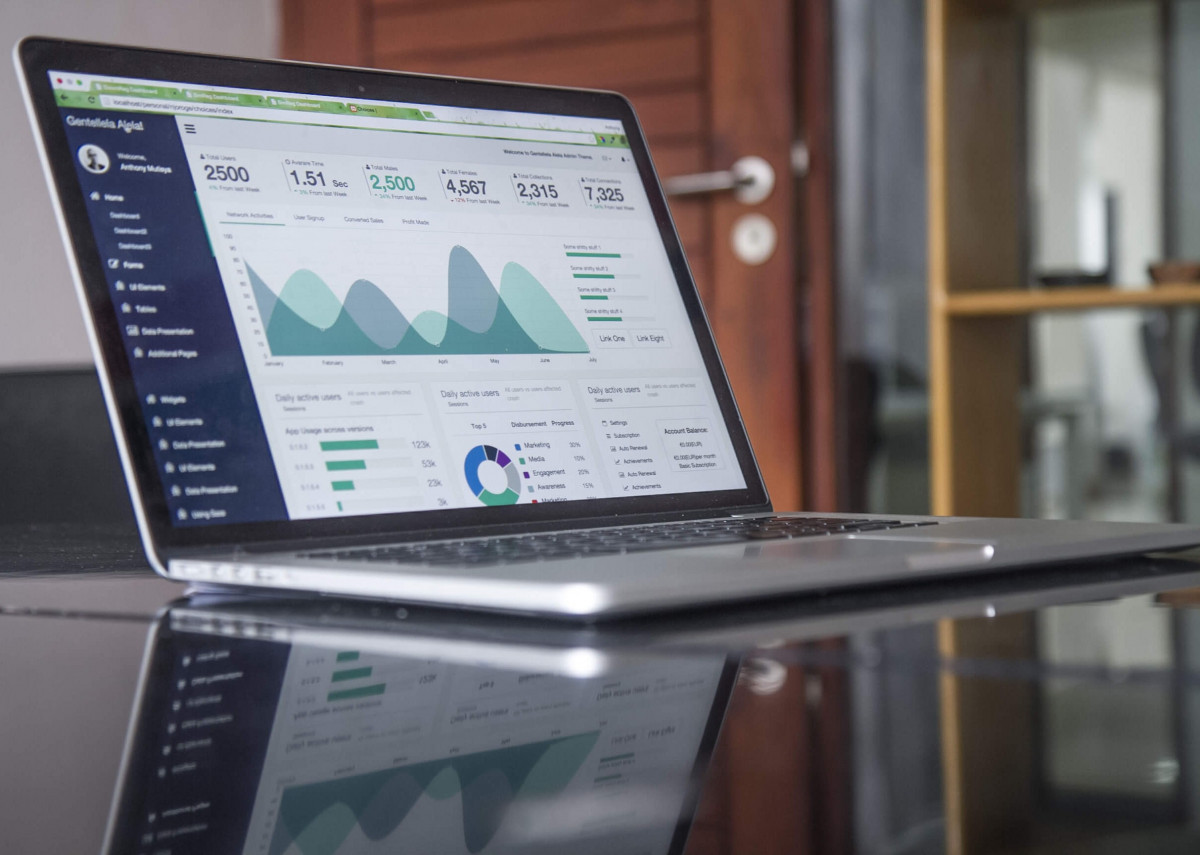# Google Sheets Tips and Tricks# Google Sheets Tips and Tricks

Well I barely use spreadsheets anymore and when I do they are all Google Sheets because the collaboration works so much better. As an old 1-2-3 and then Excel junky (Excel rules still!), there are so many things that are just a little bit different, but if you are creating models, here are some tips and tricks:

1. Most important, don't stuff random assumptions in your model in the cells themselves, you will never find them! Instead, stick all the assumptions on a given line into a set of assumption columns. So if you have three assumptions for say start of revenue (probably later now that in January 2020 :-), the initial volume and then annual increase, create three columns and document it.
2. It is really nice to nicely format these assumptions in the first column, so then you will want to use the & operator. In Excel and 1-2-3, they overloaded the + to do this, but Google Sheets is more like Javascript, so if assumptions for row 11 are in say columns G, H and I, then column A might read like ='Revenue assumes start in '& G11 &' at '&H11&' grow '& I11 &' annually'
3. Also, another note is the above loses all formatting, so if you want say a nice percent sign, then use the text function, so it might be more like text(I11,'%') which will convert the value to a percent sign and text(g11, "#,###") will give you comma separate values
4. You can also use this to tell you about offsets, so if you want say year 2 to be the start rather than year 1, like in Excel, you use the offset which let's you do relative selections and you can use row() and column() to figure out where you are in the sheet. So for instance, if you want start in year 2 a specific cost, the sheet looks like a test first to see what year you are in and then to set zero or a number so for example if(column(b23)=$g23,$h23,0) which says if you are in year the column listed in g23, then that column should be \$h23.